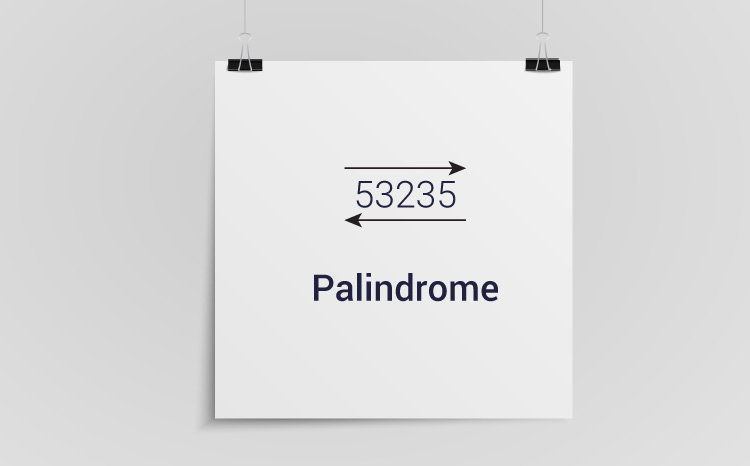# C Program to Check Whether a Number is Palindrome or Not

This program reverses an integer (entered by the user) using while loop. Then, if statement is used to check whether the reversed number is equal to the original number or not.To understand this example, you should have the knowledge of following C programming topics:

An integer is a palindrome if the reverse of that number is equal to the original number.

## Example: Program to Check Palindrome

``````#include <stdio.h>
int main()
{
int n, reversedInteger = 0, remainder, originalInteger;

printf("Enter an integer: ");
scanf("%d", &n);

originalInteger = n;

// reversed integer is stored in variable
while( n!=0 )
{
remainder = n%10;
reversedInteger = reversedInteger*10 + remainder;
n /= 10;
}

// palindrome if orignalInteger and reversedInteger are equal
if (originalInteger == reversedInteger)
printf("%d is a palindrome.", originalInteger);
else
printf("%d is not a palindrome.", originalInteger);

return 0;
}``````

Output

```Enter an integer: 1001
1001 is a palindrome.```